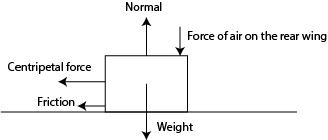# A 640kg race car can drive around a circular bend at a maximum speed of 185 km/h without...

## Question:A 640kg race car can drive around a circular bend at a maximum speed of 185 km/h without slipping. The radius of the bend is 150 m. Air flowing over the car's rear wing (see drawing) exerts a downward-pointing force of 11 kN on the car.

(a)

Draw a carefully labeled force diagram showing all the forces acting on the car.

(b)

What is the coefficient of static friction between the race track and the car's tyres?

(c)

What would be the maximum speed without slipping if the wing was removed from the car? Assume that the weight of the wing is negligible compared to the total weight of the car.

(d)

Use the low-speed approximation to calculate the kinetic energy of the car going around the bend at maximum speed (with its wing attached).

(e)

Quantify the error that you made in (d) by using the low-speed approximation.

Hint: The following expression might be useful:

{eq}\frac{1}{\sqrt{1-x}}=1+\frac{1}{2}x+\frac{3}{8}x^2+\frac{5}{16}x^3+\cdots \cdots {/eq}

## Friction:

Friction is defined as a force that opposes the motion of a particle as it slides through the surface of another object. Friction is defined as a contact force, so it will only exist when there's contact between two different surfaces

• Types of friction:We can define different types of friction. We can define static and kinetic as the most important ones. Static friction consists of the friction that opposes the motion of objects at rest (i.e in static equilibrium), while kinetic friction opposes the motion of objects that are in motion (i.e. unbalanced forces).
• Friction formula: We can determine the friction acting on an object using the following formula.

$$f_r=\mu F_n$$

• Newton's 2nd law: Newton's 2nd law states that an object will accelerate with a magnitude that is proportional to the force applied and inversely proportional to the mass of the object, hence the net force acting on a system will be proportional to both mass and acceleration. The formula to calculate the net force will be the following:

$$F=ma$$

Become a Study.com member to unlock this answer! Create your account

#### Part a:#### Part b:

As the centripetal force will be the one giving the car the ability to move in a circle, we can state the following...Friction: Definition and Types

from

Chapter 7 / Lesson 5
39K

You experience friction all the time, and you should be glad you do! Friction helps keep stationary objects in place as well as slow moving objects down as they slide across a surface. This lesson identifies what friction is and explains the two ways we find this force on earth.